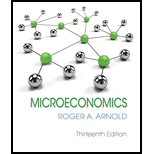# “The firm’s entire marginal cost curve is its short-run supply curve.” Is the preceding statement true or false? Explain your answer.### Microeconomics

13th Edition
Roger A. Arnold
Publisher: Cengage Learning
ISBN: 9781337617406### Microeconomics

13th Edition
Roger A. Arnold
Publisher: Cengage Learning
ISBN: 9781337617406

#### Solutions

Chapter
Section
Chapter 9, Problem 1QP
Textbook Problem

## “The firm’s entire marginal cost curve is its short-run supply curve.” Is the preceding statement true or false? Explain your answer.

Expert Solution
To determine

Whether the given statement is true or false.

### Explanation of Solution

Technically, the statement the “firm’s entire marginal cost curve is its short-run supply curve”, which is not true. The marginal cost curve and its short-run supply curve are different. The short-run supply curve is the firm’s entire marginal cost curve only when the price of the goods is above the minimum point of the average cost curve. A firm must cover its variable cost in the short run.

Economics Concept Introduction

Marginal cost (MC): Marginal cost refers to the amount of additional costs incurred in the process of increasing one more unit of output. Marginal cost curve shows the additional cost for the different levels of output.

Short-run supply curve: In the short run, it is a curve that shows the relationship between the price level in the economy and the supply in the economy by the firms.

### Want to see more full solutions like this?

Subscribe now to access step-by-step solutions to millions of textbook problems written by subject matter experts!

Get Solutions

### Want to see more full solutions like this?

Subscribe now to access step-by-step solutions to millions of textbook problems written by subject matter experts!

Get Solutions

Find more solutions based on key concepts
Show solutions
Classify consumer products

MKTG 12:STUDENT ED.-TEXT

What is the relationship between supply and demand?

Foundations of Business (MindTap Course List)

PREFERRED STOCK VALUATION Ezzell Corporation issued perpetual preferred stock with a 10% annual dividend. The s...

Fundamentals of Financial Management, Concise Edition (with Thomson ONE - Business School Edition, 1 term (6 months) Printed Access Card) (MindTap Course List)